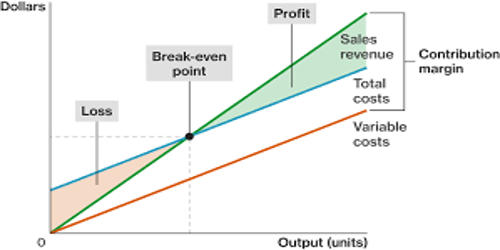Accounting

# Concept of Contribution MarginConcept of Contribution Margin

The contribution margin is the excess of sales revenue over variable costs. It is computed as the selling price per unit, minus the variable cost per unit. Thus, contribution margin is the balance of the sales revenue left after recovering variable expenses and thus is available to recover fixed expenses and to realize profits for the period. It provides one way to show the profit potential of a particular product offered by a company and shows the portion of sales that helps to cover the company’s fixed costs. Therefore contribution margin is used first to cover the fixed expenses, and then whatever remains after the recovery of the fixed expenses goes towards profits. It is a product’s price minus all associated variable costs, resulting in the incremental profit earned for each unit sold. If the contribution margin is not sufficient to cover the fixed expenses, then a loss occurs for the period. It is an important input in the calculation of breakeven point, i.e. the sales level (in units and/or dollars) at which a company makes zero profit.

Further, the concept can be used to decide which of several products to sell if they use a common bottleneck resource so that the product with the highest contribution margin is given preference.

Contribution Margin = Net Sales Revenue – Variable Costs

The total contribution margin is the total sales revenue less total variable costs. It is called the contribution margin because whatever is left after covering the variable costs contributes to pay for fixed costs and to earn a profit. It represents the incremental money generated for each product/unit sold after deducting the variable portion of the firm’s costs. If the contribution margin is not sufficient to cover the fixed costs, then the firm suffers from a loss. If the contribution margin at a particular price point is excessively low or negative, it would be unwise to continue selling a product at that price. It is expressed either as total contribution margin, contribution margin per unit, or contribution margin ratio. For example, if the price of your product is \$20 and the unit variable cost is \$4, then the unit contribution margin is \$16.

The total contribution margin generated by an entity represents the total earnings available to pay for fixed expenses and to generate a profit. The contribution margin per unit (CMPU) is the selling price per unit less(SPPU) fewer variable costs per unit (VCPU).

Therefore, CMPU= SPPU- VCPU